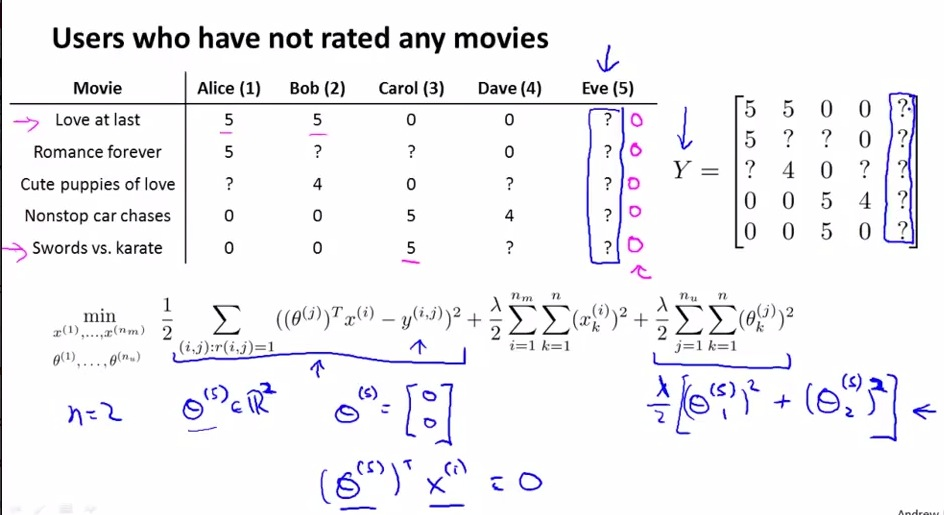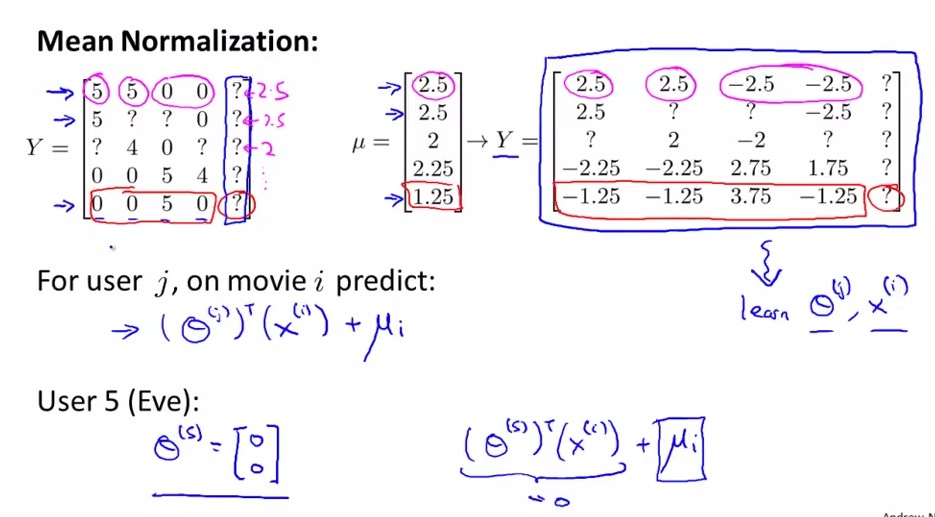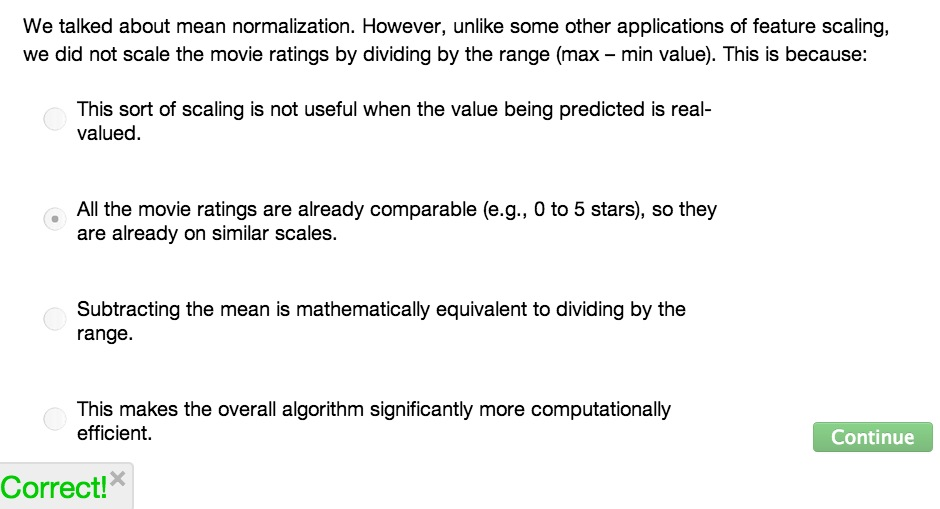• By now, we already have quite a grasp about the Recommendation System and Collaboration Filtering algoirthm
• Now we learn how the Mean Normalization to make the algorithm even more powerful• Sometimes we face users with no rating about any product. For the movies example, the user 5 doesn't rating any movies at all
• So what are we going to do? In the algorithm, suppose we insert users 5
• Thetas5 try to learn two features ( Not n+1, just n)
• Then from the equation, we know that the cost function is nullified. Because the cost function itself is trying to learn based on the user's rating (which he doesn't rate any at all), then the cost function equals zero
• Then we left of the Regularization term. And we also know the regularization trying to makes vector thetas5 to become smaller,  but theta5 already zero.
• In other words, we have no data at all.
• If we insert theta5 which equals zero to calculation for predicting user's rating, then we have zero prediction
• This means that the user rate any movies zeros. For this, we can't recommend any movies at all, because the distance is huge, and it's hard to find the relatedness about other movies
• Use Mean Normalization try to address this problem• Here's  what we are going to do
• First, we are going to compute mean for each of row in matrix Y
• That is, we trying to average the rating of each movie
• Question mark not count! So total of average minus question mark
• Then, substract each of row element with mean to produce new matrix Y
• This way, the average of row from new matrix Y equals zero
• with this, like saying "this is dataset from users", new matrix Y treated as dataset for Collaborative Filtering algorithm(instead of original matrix Y)
• then we add mean i for every movie i that user j rated, just like calculation above(prediction ratings)
• This way we try to mean normalize the rating of all movies
• Specifically for user 5, then prediction rating equals zero (because theta5 equals zero) but we're adding mean normalization i
• So even if Eve doesn't rate any movies at all, she left of the prediction of all the movie
• So this way we try to normalize the column equals zero
• From this formula, theoretically we can also do the same thing to the mean row matrix Y, that is trying to predict the rating of movie that never been rated at all
• This works for some problem, but for movies examples, this might not be a good case. Why we are trying to recommend movies that never been rated of any users? We can just drop the movies. If it good, then at the very least, it should get rating.
• Don't recommend movies to users with no rating, this also have to keep in mind for similar problem
• Taking the focus on user with don't rate at all is more important with movies that has no rating at all• RS doesn't divided by m.

• In Summary, we can use Mean Normalization for pre-processing to make Collaboration Filtering Algorithm more powerful